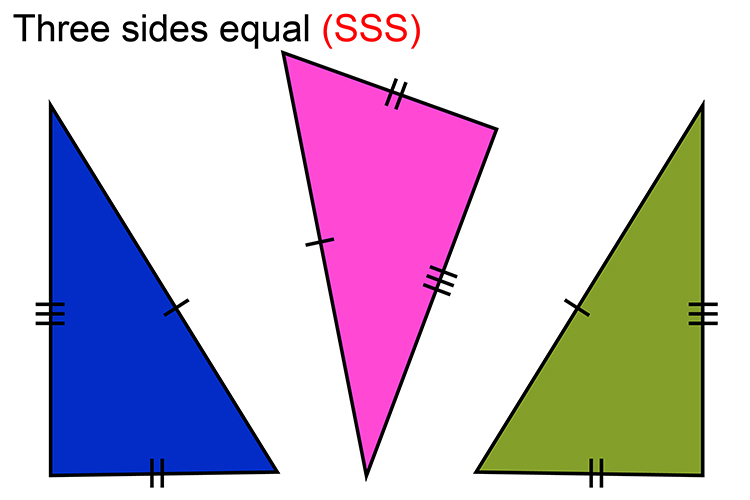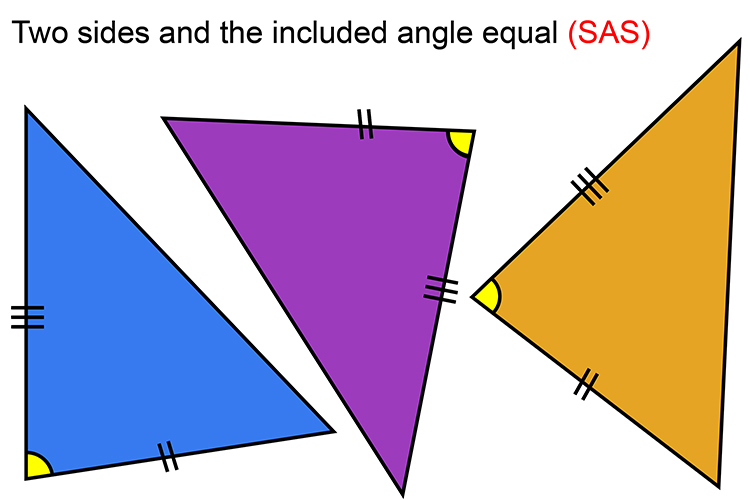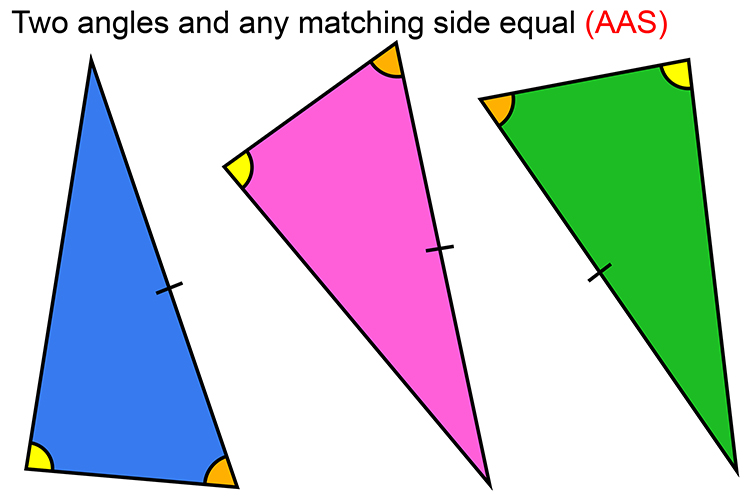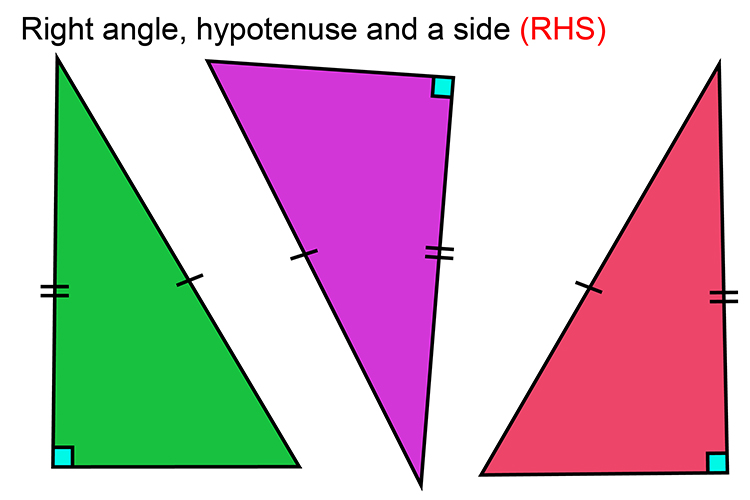# Congruency rules

Congruency rules are useful when dealing with triangles, as below. If two triangles fit one of the rules, then they are congruent.If the three sides of one triangle have the same lengths as three sides of another, then they will be congruent.If two sides of one triangle have the same length as two sides of another, and the angle between the two sides is the same on both triangles, then they will be congruent.If two angles within one triangle are the same as two angles within another, and one of the sides matches in length on both triangles, then they will be congruent.If two right angle triangles have the same length hypotenuse and one other side, they will be congruent.